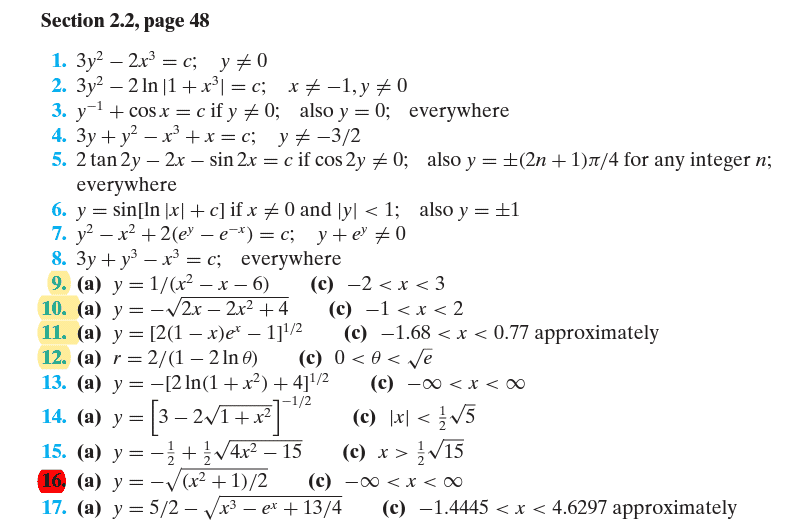# B.2.2.2 solve DE ....separate variables

• MHB
• karush
So, what Romsek is saying is that you can "multiply" both sides by dx, and then use the properties of derivatives to get y'= y(x+h) and dy= f'(x)dx.f

#### karush

Gold Member
MHB#2

$y'= \dfrac{x^2}{y(1+x^3)}$

Separate y dy =\dfrac{x^2}{(1+x^3)...

ok i tried to get the book ans but someahere derailed
why is =c in the answer

$$\displaystyle y^\prime = \dfrac{x^2}{y(1+x^3)}\\~\\ y dy = \dfrac{x^2}{1+x^3}dx\\~\\ \dfrac 1 2 y^2 = \dfrac{1}{3} \log \left(x^3+1\right) + C\\~\\ y^2 = \dfrac 2 3 \log(x^3+1) + C~\text{(note the constant absorbs multiplication by other constants)}\\~\\ y = \pm \left(\dfrac 2 3 \log(x^3+1) + C\right)^{1/2} = \pm \sqrt{\dfrac 2 3} \left(\log(x^3+1)+C\right)^{1/2}\\~\\ \text{C is in the answer because there is a whole family of curves parameterized by C that are a solution to this diff eq.}\\~\\ \text{You can select a specific curve by specifying initial conditions.}$$

ok i don't see how you can just
put dx in separations

great help tho appreciate the steps

ok i don't see how you can just
put dx in separations
Huh? What step are you not understanding?

-Dan

the second step the dy was already there the dx wasn't

$$\displaystyle y^\prime = \dfrac{dy}{dx}$$

so just "multiply" both sides by $$\displaystyle dx$$

Have you read on how to solve separable diff eqs?

Before you take Differential Equations you need to know Calculus really well! I think you need more practice in Calculus.

You say " the dy was already there the dx wasn't ".
Strictly speaking neither was in the original equation- it had y'. But I am sure you understand that y' and dy/dx are just different notations for the derivative of y with respect to x.

Romsek said "just multiply both sides by dx". Now, Strictly speaking "dy/dx" is NOT a fraction, it is rather the limit of fractions: $\lim_{h\to 0}\frac{y(x+h)- y(x)}{h}$. But it can be "treated like a fraction". To make use of that we define (typically in Calculus 3) the "differentials" dy and dx separately from dy/dx such that dy= y' dx. That allows us to treat dy/dx as if it were a fraction and say if dy/dx= f'(x) then dy= f'(x)dx.